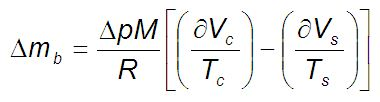# Gravimetric Measurement of Hydrogen Sorption by Materials

The gravimetric technique is one of the most common methods of determining sorption mechanisms using a microbalance. It also measures weight change to determine sorption of a material.

This article demonstrates the practical considerations to ensure measurement accuracy when performing gravimetric measurements.

## Practical Considerations for Gravimetric Measurements

The following are some of the parameters that affect the accuracy of the gravimetric measurement:

### Microbalance Stability

Accurate gravimetric measurements require a stable weight measurement. Instruments which have intrinsic long term stability are preferred over those which need secondary corrections, such as re-zeroing during measurement. In order to maintain weight calibration across the measurement, thermosetting of microbalance and instrument is needed.

It is necessary to check for unexpected hysteresis in sorption-desorption isotherms, and blank scans can be performed with a null sample.

### Temperature and Pressure Stability

Instabilities in temperature or pressure may result in corresponding oscillations in the kinetic data. Gas consumption by the sample will lead to a decrease in pressure, but active regulation can maintain a constant applied pressure.

Active feedback temperature control can compensate for any changes in temperature eg, due to changes in the lab ambient temperature or sample effects. It is therefore useful to review the full raw data as well as equilibrium isotherms.

### Buoyancy correction

Buoyancy correction is necessary for gravimetric measurements due to the displacement of a volume of gas by the sample. Although symmetric systems compensate for this to a certain extent, the buoyancy correction still needs to be performed using the following equation, where correction, Δmb, for an ideal gas in µg is given by:where Δp is the pressure change in bar, M is the molar mass, T is the temperature, R is the gas constant, δVc is the volume displaced on the counterweight side and δVs is the volume displaced on the sample side.

For non-ideal gases, buoyancy correction is carried out using the equation of state (EOS) with the compressibility factor, Z. There are many EOS with varying levels of accuracy. Hence, it is necessary to choose the appropriate EOS for a specific application.

The buoyancy-corrected data provides excess uptake, and the conversion of excess uptake into absolute uptake is carried out using the sorbed phase density. Blank (unloaded) measurements allow verification of the correction in the absence of sample.

### Sample Size

The ability to measure “small” samples using a microbalance depends on the expected magnitude of the sorption uptake and the instrument’s efficiency. Considering signal to noise parameter and ensuring optimization of the instrument for research scale samples are critical in gravimetric measurements.## Citations

• APA

Hiden Isochema. (2019, July 18). Gravimetric Measurement of Hydrogen Sorption by Materials. AZoM. Retrieved on October 26, 2020 from https://www.azom.com/article.aspx?ArticleID=12004.

• MLA

Hiden Isochema. "Gravimetric Measurement of Hydrogen Sorption by Materials". AZoM. 26 October 2020. <https://www.azom.com/article.aspx?ArticleID=12004>.

• Chicago

Hiden Isochema. "Gravimetric Measurement of Hydrogen Sorption by Materials". AZoM. https://www.azom.com/article.aspx?ArticleID=12004. (accessed October 26, 2020).

• Harvard

Hiden Isochema. 2019. Gravimetric Measurement of Hydrogen Sorption by Materials. AZoM, viewed 26 October 2020, https://www.azom.com/article.aspx?ArticleID=12004.